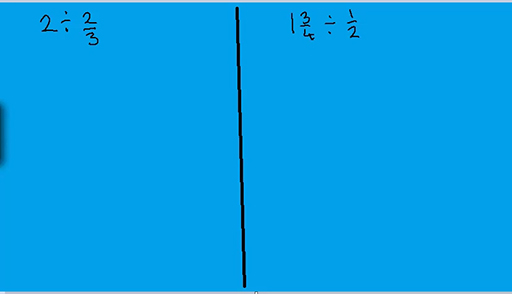Teaching mathematics

Start this free course now. Just create an account and sign in. Enrol and complete the course for a free statement of participation or digital badge if available.

Free course

# 2.5 Dividing fractions

The standard procedure for dividing by a fraction is one of those where learners can get incredibly confused. ‘Turn it upside-down and multiply’ is the common mantra but which fraction (if there are two) and why does it work?

Division is the most difficult of the arithmetic functions, even for whole numbers. It can help to go back to remembering what division means. The grouping method of division is most useful when dealing with fraction division. Watch Video 3 for some useful approaches to teaching division with fractions.Skip transcript: Video 3 Dividing with fractions

#### Transcript: Video 3 Dividing with fractions

INSTRUCTOR:
I'm going to look at the problem 2 divided by 2/3. What does this mean? One understanding of division is that the problem is asking, how many 2/3's are there in 2?
I can work this out by counting up in 2/3's and seeing where I get 2. So if I've got one 2/3 and then add on another 2/3 to that, I'd have 4/3. And if I add on another one, I'll get 6/3.
Now, 6/3 is 2. So the answer to the question 2 divided by 2/3 is 3.
This question was easily solved by counting up in 2/3's, but some questions are not so easy. Consider this question, 1 and 3/4 divided by 1/2, which we could say as, how many 1/2's are there in 1 and 3/4?
If I count up in 1/2's, I'd get 1/2, 1, 1 and 1/2, 2, and so forth. So I can see that 1 and 3/4 is in between 1 and 1/2 and 2. So I expect my answer to be a bit bigger than 3.
I'm going to look at this by drawing a diagram. I'm going to draw 1 and 3/4 circles. So that's one circle and then 3/4 circles.
OK. And I'm going to split those up into halves. So the whole circle will have 1/2 in it and another 1/2. And then this 3/4 circle will be 1/2 here, and then we've got a quarter of a circle here.
So I can see from this that I've got three halves, and I've also got the quarter. Now, that quarter is a quarter of a circle. But we're actually looking at 1/2's. So when we're looking at that, we're actually looking at a half of a half. This quarter is a half of a half.
So we've actually got one, two, three halves, 3/2, and then a half of a half. So 1 and 3/4 divided by 1/2 is 3 and 1/2.
I can also choose to do these calculations using the well-known method of turn it upside down and multiply. So it's a nice, quick, and easy way to do it. But you do have to remember the procedure and how it works.
And it's OK if only the procedure is done properly. And what a lot of learners do is think that it's both the numbers that have to be turned upside down, which obviously is the problem here.
So it's actually the second number which is turned upside down and multiplied. If we look at this one here, so 2 divided by 2/3 is the same as 2 multiplied by 3/2.
OK. So 2 lots of 3/2 is 6/2, which is 3. And in this case, 1 and 3/4 divided by 1/2 is the same as 1 and 3/4 times 2/1, 2/1 being 2. That basically means you're doubling it, so that's 3 and 1/2.
What you're doing when you turn it upside down and multiply is you are multiplying by the reciprocal of the second number. In other words, the number which is 1 divided by it. So 2/3 and 3/2, they actually are reciprocals. Because if you multiply them together, you would get 1.
And similarly here, 1/2 and 2/1 are reciprocals, because if you multiply them together, you get 1. So this is what's happening here. You're multiplying by the reciprocal, which is, as it were, equivalent to dividing by the original number.
End transcript: Video 3 Dividing with fractions
Video 3 Dividing with fractions
Interactive feature not available in single page view (see it in standard view).

The ‘turn it upside-down and multiply’ method works because turning a fraction upside-down produces its multiplicative inverse. Fractions are unlike the integers in that they have a multiplicative inverse which can be used in fraction division because multiplying is also the inverse of dividing.

In the next section you will meet the mathematical cakes model again as a way to help learners to find percentages of amounts.

TM_1